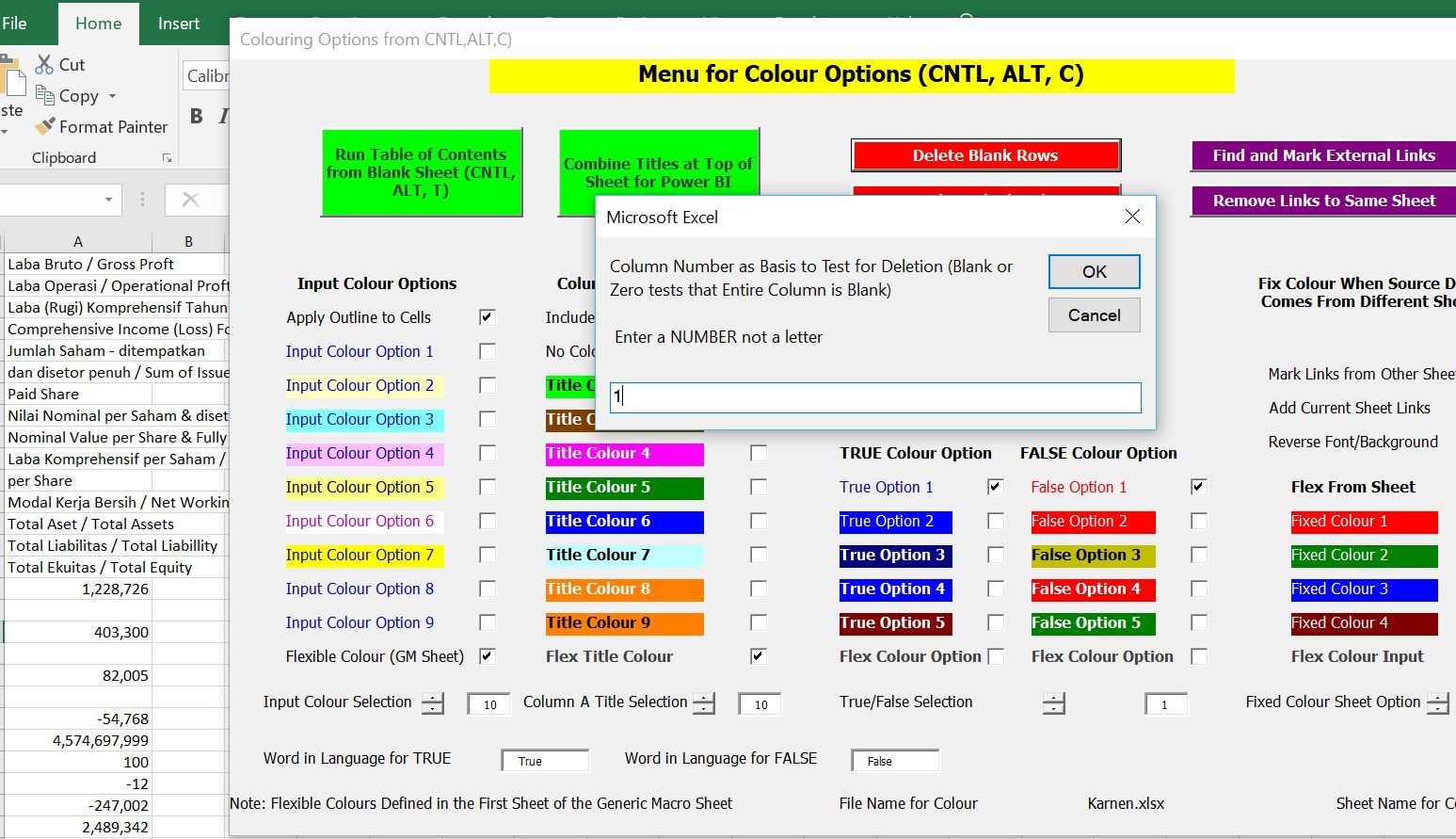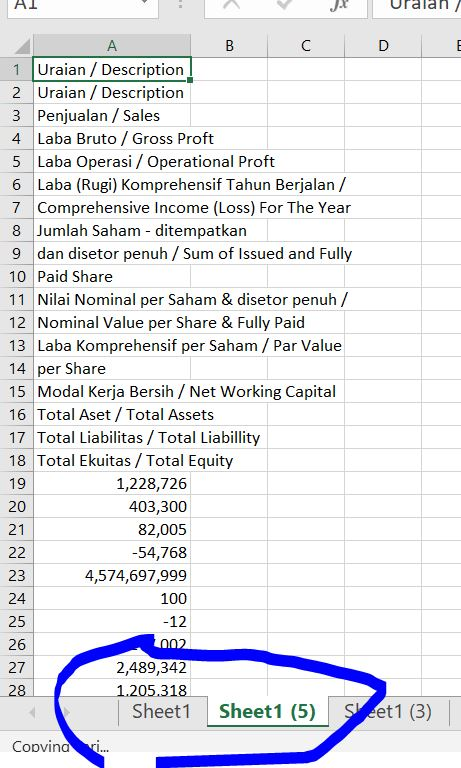# Delete Blank Row

This page demonstrates how you can use and create a macro that deletes blank rows in excel.  In working with this program, you need to define a column that is used to test for blanks.  A loop works through the rows for the selected column.  When a blank is found it is deleted.  You should work backwards from the bottom of the sheet.  A new sheet is make in case there is a problem with the macro.

## Using the Delete Rows Macro in Generic Macros

The sheet below demonstrates an example of a file that has some blanks.  If you want to delete the rows that are blank you can use the generic macro file.To remove blank rows, I created a macro in the generic macros file.  So, open the generic macro file.  Then press the CNTL, ALT, C sequence to get the colouring menu.  On this menu there is a option for deleting blank rows as shown in the screenshot below.  Sorry about the crude circle.After you press the delete rows button, a input box message appears.  In this box, you enter the column number to test for deleting rows.  This means that if the column you selected has a blank, the row will be deleted.  The screenshot below demonstrates how you enter the column number (not the column letter).After you enter the column number, the rows are deleted and the results are put in a new sheet (in case you do something wrong and to much is deleted).  The manner in which the blank rows are deleted is demonstrated in the screenshot below.  Notice that sheet1 — the place where the original rows were read in is now sheet1(5) — this is a new sheet with the deleted blank rows.  The original sheet is still there.## Technical Details for Writing Your Own Macro

The VBA code below demonstrates how you can do this yourself.  You need to find the number of rows and work backward with a for loop.   A macro for deleting rows and deleting columns is presented.

```.

Sub x_Delete_rows()

Dim col_test As Single

x_find_rows
x_find_cols

On Error GoTo set_zero:
col_test = InputBox("Column Number as Basis to Test for Deletion (Blank or Zero tests that Entire Column is Blank)" _
& Chr(13) & Chr(13) & " Enter a NUMBER not a letter")
GoTo skip:
set_zero:
col_test = 0
skip:

copy_sheet

If col_test = zero Then
start_col = 1
end_col = max_col
Else
start_col = col_test
end_col = col_test
End If

' MsgBox " Maximum Rows " & max_row & " Maximum Columns " & max_col

For delete_row = max_row To 1 Step -1 ' work through rows from end
keep_row = False ' keep rows set to false (meaning delete)
Cells(delete_row, 1).Select

For col = start_col To end_col ' work around cols check if anything there

If WorksheetFunction.IsNumber(Cells(delete_row, col)) = True _
Or WorksheetFunction.IsText(Cells(delete_row, col)) = True Then
keep_row = True
End If
Next col

If keep_row = False Then ' delete row if you havent found anything
Selection.EntireRow.Delete
End If

Next delete_row

End Sub

Sub x_Delete_cols()
'
x_find_rows ' find rows and cols from this function
x_find_cols

' MsgBox " Maximum Rows " & max_row & " Maximum Columns " & max_col

For delete_col = max_col To 1 Step -1 ' key is to work backwards
keep_col = False
Cells(1, delete_col).Select
For row = 1 To max_row
If WorksheetFunction.IsNumber(Cells(row, delete_col)) = True _
Or WorksheetFunction.IsText(Cells(row, delete_col)) = True Then
keep_col = True
End If
Next row

If keep_col = False Then ' After finding blank colum, delete
Selection.EntireColumn.Delete
End If

Next delete_col

End Sub

```